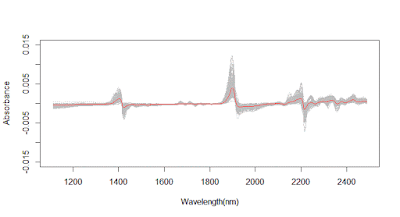Exercises

Tutorials with Resemble (Part 1)

Tutorials with Resemble (Part 2)

Tutorials with Resemble (Part 3)
3.1: Practice plotting the average spectrum.
matplot(wavelength_der,t(der.Xr),type="l",col="grey",
xlab="Wavelength(nm)",ylab="Absorbance",
ylim=c(-0.015,0.015))
par(new=TRUE)
matplot(wavelength_der,center,type="l",col="red",
xlab="Wavelength(nm)",ylab="Absorbance",
ylim=c(-0.015,0.015))The average spectrum is used to subtract it from every spectrum in the training or validation set, this is known as “Center”, this way we can see better the source of variances in the sample sets.
der.Xr.c<-scale(der.Xr,center=TRUE,scale=FALSE)
matplot(wavelength_der,t(der.Xr.c),type="l",col="blue",
xlab="Wavelength(nm)")

﻿